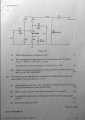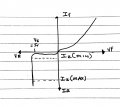# Transistor / Zener Diode Exam Question. Help Needed.

#### rq89

Joined Mar 11, 2015
9
My lecturer is going to be going over this question next week but I wanted to get a start on it in order to fully understand it. Here is what I think I know so far and any assistance would be great.

(a) (i) Zener Diode - voltage regulator
(ii) ?
(iii) See attached sketch

(b) (i) ?
(ii) C1 - Coupling capacitor to connect a.c. supply from input to base
C2 - Coupling capacitor to connect to output circuit
C3 - Decouples any a.c. variation on R4 allowing gain?

(iii) Av = - (R3/R4)

(iv) ?#### rq89

Joined Mar 11, 2015
9
R4 = Ve/Ie = 0.5v/5mA = 100 ohms

Vc is approximately half supply voltage but I'm not sure if I use 12v or 18v and if i take into account the 10v the zener will use at this point.

VR3 = Ie x R3
Vc = Vcc-VR3

Assumptions:
Ib = 0A
base/emitter voltage = 0.6v
Ie=Ic

Last edited:

#### WBahn

Joined Mar 31, 2012
26,398
For question (a)(ii), what is the maximum current that can be allowed in that zener diode without exceeding the specs? If all of the current from the power supply goes through the zener, what value does R5 need to be in order to ensure that the zener does not exceed specs?

Why does your diagram show Vz being 5V? Does that agree with the data given in the prior part?

#### rq89

Joined Mar 11, 2015
9
For question (a)(ii), what is the maximum current that can be allowed in that zener diode without exceeding the specs? If all of the current from the power supply goes through the zener, what value does R5 need to be in order to ensure that the zener does not exceed specs?

Why does your diagram show Vz being 5V? Does that agree with the data given in the prior part?
Apologies. I should have stated that this was a diagram I had done for a previous circuit. Should be 10v for this one.

#### rq89

Joined Mar 11, 2015
9
Pz(max) = 1.3w
Vz = 10v
Iz(max) = Pz(max)/Vz = 130mA

IR5 = Vs-Vz/R5
130mA = 18v-10v/R5
R5 = 8v/130mA = 61.5ohms

#### WBahn

Joined Mar 31, 2012
26,398
Thank you for tracking your units!!!

You do need to watch out for order of operations, though. Vs-Vz/R5 is the same as (Vs)-(Vz/R5), which is not correct (and notice that the units don't work out).

So you've got your R5. Now what is the current budget that you have to work with for the rest of the circuit?